## Prime Number Theorem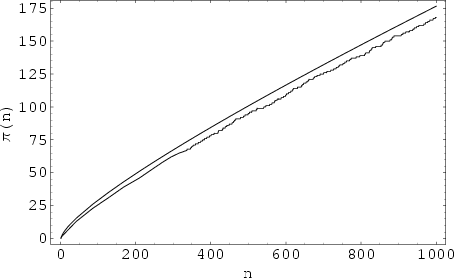The theorem giving an asymptotic form for the Prime Counting Function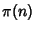for number of Primes less than some Integer. Legendre(1808) suggested that, for large,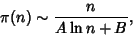(1)

with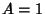and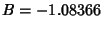(where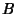is sometimes called Legendre's Constant), a formula which is correct in the leading term only (Wagon 1991, pp. 28-29). In 1791, Gaußbecame the first to suggest instead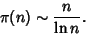(2)

Gaußlater refined his estimate to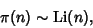(3)

where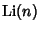is the Logarithmic Integral. This function has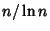as the leading term and has been shown to be a better estimate thanalone. The statement (3) is often known as the'' prime number theorem and was proved independently by Hadamardand Vallée Poussinin 1896. A plot of(lower curve) andis shown above for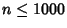.

For small, it has been checked and always found that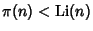. However, Skewes proved that the first crossing of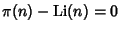occurs before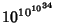(the Skewes Number). The upper bound for the crossing has subsequently been reduced to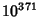. Littlewood (1914) proved that the Inequality reverses infinitely often for sufficiently large(Ball and Coxeter 1987). Lehman (1966) proved that at least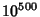reversals occur for numbers with 1166 or 1167 Decimal Digits.

Chebyshev (Rubinstein and Sarnak 1994) put limits on the Ratio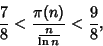(4)

and showed that if the Limit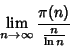(5)

existed, then it would be 1. This is, in fact, the prime number theorem.

Hadamard and Vallée Poussin proved the prime number theorem by showing that the Riemann Zeta Function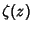has no zeros of the form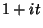(Smith 1994, p. 128). In particular, Vallée Poussin showed that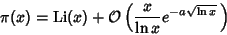(6)

for some constant. A simplified proof was found by Selberg and Erdös (1949) (Ball and Coxeter 1987, p. 63).

Riemannestimated the Prime Counting Function with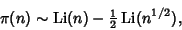(7)

which is a better approximation thanfor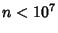. Riemann (1859) also suggested the Riemann Function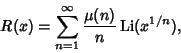(8)

where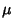is the Möbius Function (Wagon 1991, p. 29). An even better approximation for small(by a factor of 10 for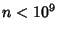) is the Gram Series.

The prime number theorem is equivalent to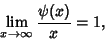(9)

where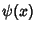is the Summatory Mangoldt Function.

The Riemann Hypothesis is equivalent to the assertion that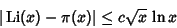(10)

for some value of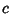(Ingham 1932, Ball and Coxeter 1987). Some limits obtained without assuming the Riemann Hypothesis are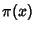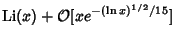(11)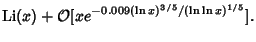(12)

Ramanujanshowed that for sufficiently large,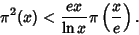(13)

The largest known Prime for which the inequality fails is 38,358,837,677 (Berndt 1994, pp. 112-113). The related inequality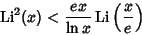(14)

is true for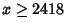(Berndt 1994, p. 114).

See also Bertrand's Postulate, Dirichlet's Theorem, Gram Series, Prime Counting Function, Riemann's Formula, Riemann Function, Riemann-Mangoldt Function, Riemann Weighted Prime-Power Counting Function, Skewes Number

References

Ball, W. W. R. and Coxeter, H. S. M. Mathematical Recreations and Essays, 13th ed. New York: Dover, pp. 62-64, 1987.

Berndt, B. C. Ramanujan's Notebooks, Part IV. New York: Springer-Verlag, 1994.

Courant, R. and Robbins, H. The Prime Number Theorem.'' §1.2c in Supplement to Ch. 1 in What is Mathematics?: An Elementary Approach to Ideas and Methods, 2nd ed. Oxford, England: Oxford University Press, pp. 27-30, 1996.

Davenport, H. Prime Number Theorem.'' Ch. 18 in Multiplicative Number Theory, 2nd ed. New York: Springer-Verlag, pp. 111-114, 1980.

de la Vallée Poussin, C.-J. Recherches analytiques la théorie des nombres premiers.'' Ann. Soc. scient. Bruxelles 20, 183-256, 1896.

Hadamard, J. Sur la distribution des zéros de la fonction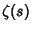et ses conséquences arithmétiques (').'' Bull. Soc. math. France 24, 199-220, 1896.

Hardy, G. H. and Wright, E. M. Statement of the Prime Number Theorem.'' §1.8 in An Introduction to the Theory of Numbers, 5th ed. Oxford, England: Clarendon Press, pp. 9-10, 1979.

Ingham, A. E. The Distribution of Prime Numbers. London: Cambridge University Press, p. 83, 1932.

Legendre, A. M. Essai sur la Théorie des Nombres. Paris: Duprat, 1808.

Lehman, R. S. On the Difference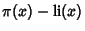.'' Acta Arith. 11, 397-410, 1966.

Littlewood, J. E. Sur les distribution des nombres premiers.'' C. R. Acad. Sci. Paris 158, 1869-1872, 1914.

Nagell, T. The Prime Number Theorem.'' Ch. 8 in Introduction to Number Theory. New York: Wiley, 1951.

Riemann, G. F. B. Über die Anzahl der Primzahlen unter einer gegebenen Grösse.'' Monatsber. Königl. Preuss. Akad. Wiss. Berlin, 671, 1859.

Rubinstein, M. and Sarnak, P. Chebyshev's Bias.'' Experimental Math. 3, 173-197, 1994.

Selberg, A. and Erdös, P. An Elementary Proof of the Prime Number Theorem.'' Ann. Math. 50, 305-313, 1949.

Shanks, D. The Prime Number Theorem.'' §1.6 in Solved and Unsolved Problems in Number Theory, 4th ed. New York: Chelsea, pp. 15-17, 1993.

Smith, D. E. A Source Book in Mathematics. New York: Dover, 1994.

Wagon, S. Mathematica in Action. New York: W. H. Freeman, pp. 25-35, 1991.